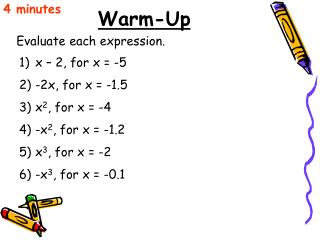Download PresentationWarm-Up

# Warm-Up - PowerPoint PPT Presentation

4 minutes. Warm-Up. Evaluate each expression. x – 2, for x = -5 -2x, for x = -1.5 x 2 , for x = -4 -x 2 , for x = -1.2 x 3 , for x = -2 -x 3 , for x = -0.1. 2.3 Intro to Functions. Objectives: State the domain and range of a relation, and tell whether it is a functionI am the owner, or an agent authorized to act on behalf of the owner, of the copyrighted work described.
Download Presentation## Warm-Up

Download Policy: Content on the Website is provided to you AS IS for your information and personal use and may not be sold / licensed / shared on other websites without getting consent from its author.While downloading, if for some reason you are not able to download a presentation, the publisher may have deleted the file from their server.

- - - - - - - - - - - - - - - - - - - - - - - - - - E N D - - - - - - - - - - - - - - - - - - - - - - - - - -
Presentation Transcript
1. 4 minutes Warm-Up Evaluate each expression. • x – 2, for x = -5 • -2x, for x = -1.5 • x2, for x = -4 • -x2, for x = -1.2 • x3, for x = -2 • -x3, for x = -0.1

2. 2.3 Intro to Functions Objectives: State the domain and range of a relation, and tell whether it is a function Write a function in function notation and evaluate it

3. Definition of a Function Function: each value of the first variable is paired with exactly one value of the second variable Domain: set of all possible values of the first variable Range: set of all possible values of the second variable

4. Example 1 State whether the data in each table represents y as a function of x. Explain. function not a function

5. Vertical-Line Test If every vertical line intersects a given graph at no more than one point, then the graph represents a function. function not a function

6. Definition of a Relation Relation: each value of the first variable is paired with one or more values of the second variable Domain: set of all possible values of the first variable Range: set of all possible values of the second variable

7. Example 2 State the domain and range of the relation, and state whether it is a function. { (–7, 5), (4, 12), (8, 23), (16, 8) } domain: { –7, 4, 8, 16} range: { 5, 8, 12, 23 } This is a function because each x-coordinate is paired with only one y-coordinate.

8. Function Notation If there is a correspondence between values of the domain, x, and values of the range, y, that is a function, then y = f(x), and (x,y) can be written as (x,f(x)). The variable x is called the independent variable. The variable y, or f(x) is called the dependent variable.

9. Example 3 Evaluate f(x) = –2.5x + 11, where x = –1. f(–1) = –2.5 (–1) + 11 f(–1) = 2.5 + 11 f(–1) = 13.5

10. Example 4 A gift shop sells a specialty fruit and nut mix at a cost of \$2.99 per pound. During the holiday season, you can buy as much of the mix as you like and have it packaged in a decorative tin that costs \$4.95. a) Write a linear function to model the total cost in dollars, c, of the tin containing the fruit and nut mix as a function of the number of pounds of the mix, n. c(n) = 4.95 + 2.99n b) Find the total cost of a tin that contains 1.5 pounds of the mix. c(n) = 4.95 + 2.99n c(1.5) = 4.95 + 2.99(1.5) c(1.5) = 9.44 \$9.44

11. Homework p.107 #17-49 odds